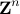# A Semidefinite Programming Method for Integer Convex Quadratic Minimization

J. Park and S. Boyd

Optimization Letters, 12(3):499–518, May 2018.

We consider the NP-hard problem of minimizing a convex quadratic function over the integer lattice. We present a simple semidefinite programming (SDP) relaxation for obtaining a nontrivial lower bound on the optimal value of the problem. By interpreting the solution to the SDP relaxation probabilistically, we obtain a randomized algorithm for finding good suboptimal solutions, and thus an upper bound on the optimal value. The effectiveness of the method is shown for numerical problem instances of various sizes.